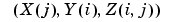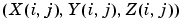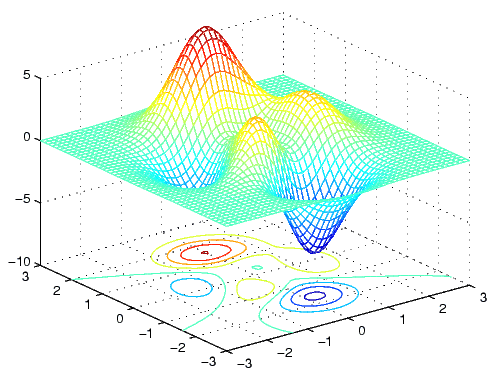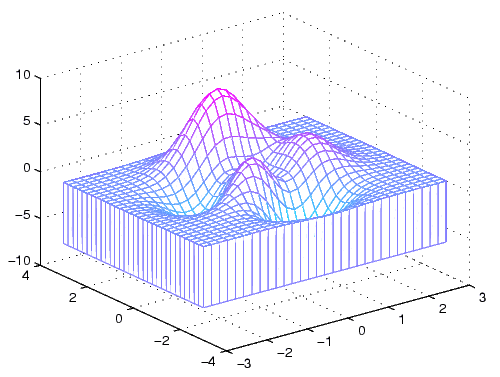MATLAB Function Referencemesh, meshc, meshz

Mesh plots

Syntax

• ```mesh(X,Y,Z)
mesh(Z)
mesh(...,C)
mesh(...,'`PropertyName`',PropertyValue,...)
mesh(axes_handles,...)
meshc(...)
meshz(...)
h =` `mesh`(`...)
h =` `meshc(...)
h =` `meshz(...)
hsurface = mesh('v6'...), = meshc('v6'...), = meshz('v6'...)
```

Description

`mesh`, `meshc`, and `meshz` create wireframe parametric surfaces specified by `X`, `Y`, and `Z`, with color specified by `C`.

```mesh(X,Y,Z) ``` draws a wireframe mesh with color determined by `Z` so color is proportional to surface height. If `X` and `Y` are vectors, `length(X) = n` and `length(Y) = m`, where `[``m,n] = size(Z)`. In this case,`` are the intersections of the wireframe grid lines; `X` and `Y` correspond to the columns and rows of `Z`, respectively. If `X` and `Y` are matrices, `````` are the intersections of the wireframe grid lines.

```mesh(Z) ``` draws a wireframe mesh using `X = 1:n` and `Y = 1:m`, where `[m,n] = size(Z)`. The height, `Z`, is a single-valued function defined over a rectangular grid. Color is proportional to surface height.

```mesh(...,C) ``` draws a wireframe mesh with color determined by matrix `C`. MATLAB performs a linear transformation on the data in `C` to obtain colors from the current colormap. If `X`, `Y`, and `Z` are matrices, they must be the same size as `C`.

```mesh(...,'PropertyName',PropertyValue,...) ``` sets the value of the specified surface property. Multiple property values can be set with a single statement.

```mesh(axes_handles,...) ``` plots into the axes with handle `axes_handle` instead of the current axes (`gca`).

```meshc(...) ``` draws a contour plot beneath the mesh.

```meshz(...) ``` draws a curtain plot (i.e., a reference plane) around the mesh.

```h = mesh(...), h = meshc(...), and h = meshz(...) ``` return a handle to a surfaceplot graphics object.

Backward Compatible Version

```hsurface = mesh('v6',...) hsurface = meshc('v6',...), and hsurface = meshc('v6',...) ``` returns the handles of surface objects instead of surfaceplot objects for compatibility with MATLAB 6.5 and earlier.

Remarks

A mesh is drawn as a surface graphics object with the viewpoint specified by `view(3)`. The face color is the same as the background color (to simulate a wireframe with hidden-surface elimination), or none when drawing a standard see-through wireframe. The current colormap determines the edge color. The `hidden` command controls the simulation of hidden-surface elimination in the mesh, and the `shading` command controls the shading model.

Examples

Produce a combination mesh and contour plot of the `peaks` surface:

• ```[X,Y] = meshgrid(-3:.125:3);
Z = peaks(X,Y);
meshc(X,Y,Z);
axis([-3 3 -3 3 -10 5])```

Generate the curtain plot for the `peaks` function:

• ```[X,Y] = meshgrid(-3:.125:3);
Z = peaks(X,Y);
meshz(X,Y,Z)```

Algorithm

The range of `X`, `Y`, and `Z`, or the current settings of the axes `XLimMode`, `YLimMode`, and `ZLimMode` properties determine the axis limits. `axis` sets these properties.

The range of `C`, or the current settings of the axes `CLim` and `CLimMode` properties (also set by the `caxis` function), determine the color scaling. The scaled color values are used as indices into the current colormap.

The mesh rendering functions produce color values by mapping the z data values (or an explicit color array) onto the current colormap. The MATLAB default behavior is to compute the color limits automatically using the minimum and maximum data values (also set using `caxis auto`). The minimum data value maps to the first color value in the colormap and the maximum data value maps to the last color value in the colormap. MATLAB performs a linear transformation on the intermediate values to map them to the current colormap.

`meshc` calls `mesh`, turns `hold` on, and then calls `contour` and positions the contour on the x-y plane. For additional control over the appearance of the contours, you can issue these commands directly. You can combine other types of graphs in this manner, for example `surf` and `pcolor` plots.

`meshc` assumes that `X` and `Y` are monotonically increasing. If `X` or `Y` is irregularly spaced, `contour3` calculates contours using a regularly spaced contour grid, then transforms the data to `X` or `Y`.

`contour`, `hidden`, `meshgrid`, `surface`, `surf`, `surfc`, `surfl`, `waterfall`
The functions axis, `caxis`, `colormap`, `hold`, `shading`, and `view` all set graphics object properties that affect `mesh`, `meshc`, and `meshz`.
For a discussion of parametric surfaces plots, refer to `surf``.`